# R Weekly Bulletin Vol – XIII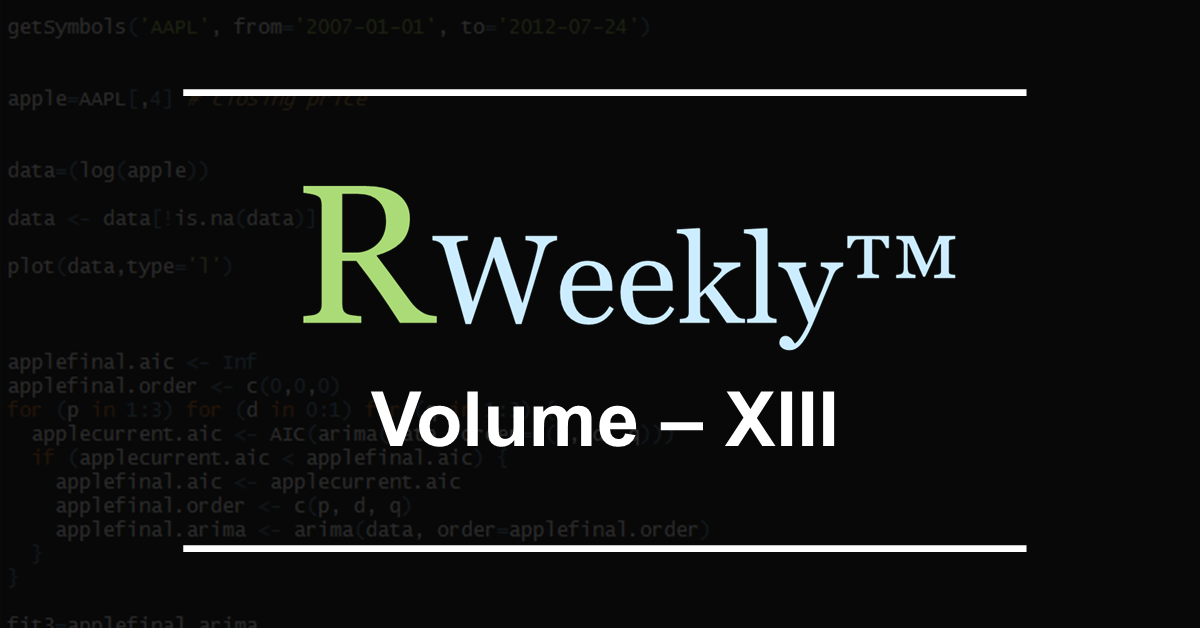This week’s R bulletin covers some interesting plotting methods. Hope you like this R weekly bulletin. Enjoy reading!

### Shortcut Keys

1. Source a file - Ctrl+Shift+O
2. Source the current document - Ctrl+Shift+S
3. Find in Files - Ctrl+Shift+F

### Problem Solving Ideas

#### Using Multiple Colors for Plotting a Variable

Plotting a variable with multiple colors allows one to discern the pattern in the plotted data easily, and can be done using the “col” argument. Remaining arguments to the plot function remains mostly the same. Let us consider an example to illustrate multi-color plotting.

Example: Here, we are sourcing the one-year data for the NSE listed MRF stock. Using the dailyReturn function from the quantmod package we compute the daily returns based on the daily closing price for the stock.

We intend to have the positive returns plotted in darkgreen color, and the negative returns in red. To do so, we create a vector called “colors” as shown below using the ifelse statement. The ifelse statement works on a vector, and we call it on the “returns” vector.

This “colors” vector is used as the value for the “col” argument in the plot function. The “type” argument in the plot function has to be kept as “h”. Remaining parameters used in the plot function are self-explanatory.

library(quantmod)
mrf = getSymbols("MRF.NS", src="yahoo", from="2017-01-01", to="2017-06-01", auto.assign=FALSE)
returns = dailyReturn(Cl(mrf))*100
date = index(mrf)
colors = ifelse(returns >= 0, "darkgreen", "red")
plot(date,returns, type="h",lwd=2, col=colors, xlab="Period", ylab="Daily returns(%)", main="MRF Daily Returns for 1H-2017")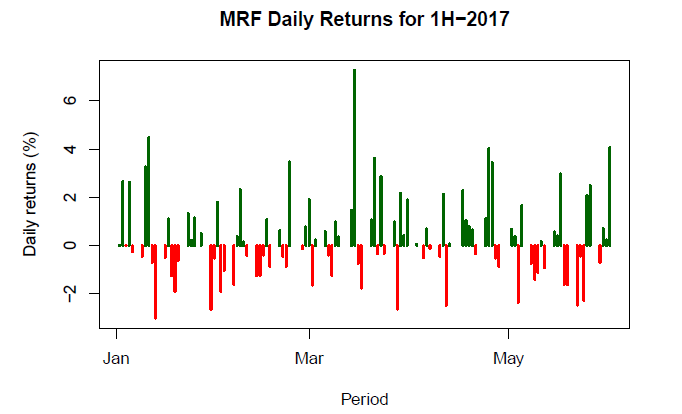#### Displaying Multiple Plotting on a Single Page

For displaying multiple plots on a single page we divide the graphics window into a matrix using the par(mfrow) function. We specify the number of rows and columns in the mfrow argument of the par function. For example, mfrow = c(2,2) will divide the space into four parts.

We then call the high-level function to plot our datasets. If we want to plot four datasets and display them separately, we will call them four times using the high-level function. The example given below illustrates the same.

Example:

library(quantmod)
ktkbank=getSymbols("KTKBANK.NS", src="yahoo", from="2016-01-01", to="2016-12-31",auto.assign=FALSE)
unionbank=getSymbols("UNIONBANK.NS",src="yahoo",from="2016-01-01",to="2016-12-31",auto.assign=FALSE)

ktkbank_close = coredata(Cl(ktkbank))
unionbank_close = coredata(Cl(unionbank))
date = index(ktkbank)

par(mfrow = c(1, 2))
plot(date, ktkbank_close, type = "l", lty = 1, pch = 19, col = "red", xlab = "Period",
ylab = "Price", main = "KTKBANK")
plot(date, unionbank_close, type = "l", lty = 1, pch = 19, col = "blue", xlab = "Period",ylab = "Price", main = "UNIONBANK")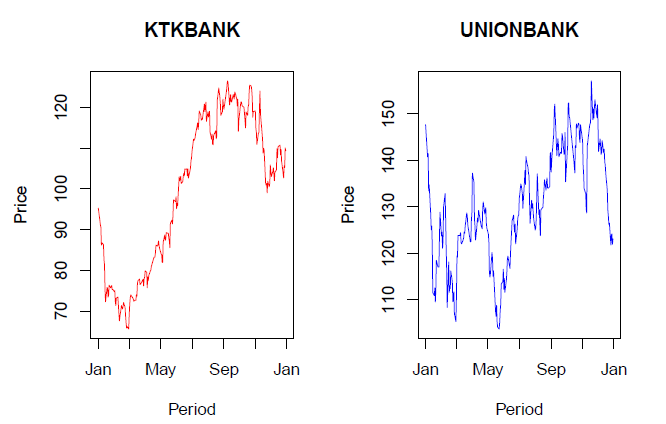#### Changing Global Parameters for Plotting

Many functions take multiple arguments. For some of these arguments, R has set default values. These default values help save time since we do not have to set a value for each and every argument of the function.

If we do not want to use a default value and set our own value for any particular argument of a function, this can be done using the par function. One can check the current default value of an argument by calling the par function with the argument name.

Example:

par("col")

 "black

In order to set a new value of one’s choice, we can set the same by calling the par function and assign the new value to the argument.

Example:

par(col="blue")

There is a long list of such arguments whose default value can be changed using the par function. Refer to the help page for the par function which lists down such arguments. Note that when you change the default value of a global parameter it will affect all your plots, not just the current one. To reset the original value, assign the argument its original value after you have done with the plotting. The example below illustrates the same:

library(quantmod)
# make a copy of current settings
original_value = par("lty")
print(original_value)

 "solid"

# set the global parameter using the par function
par(lty ="dashed")
# create a plot with the new setting
idbi=getSymbols("IDBI.NS", src="yahoo", from="2016-01-01", to="2016-12-01",auto.assign=FALSE)
high = coredata(Hi(idbi))
low = coredata(Lo(idbi))
date = index(idbi)
main = "IDBI Daily Price Chart for 2016"
plot(date, high, type = "l", pch = 19, col = "red", xlab = "Date", ylab = "Price", main = main)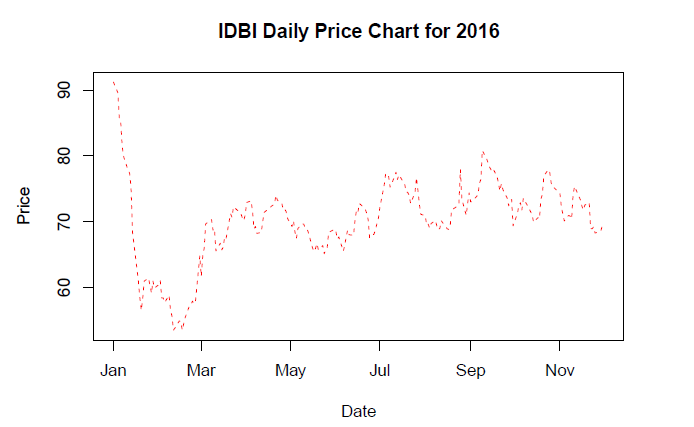# Restore the global parameter to its default value
par(lty = original_value)

### Functions Demystified

#### sapply and vapply functions

The sapply function is a user-friendly version and wrapper of lapply, it takes a list as the input and returns a vector. If the “simplify” argument is entered as “array”, the sapply function will return an array.

sapply(x, f, simplify = FALSE, USE.NAMES = FALSE) is the same as lapply(x, f)

Example:

x = list(a = 1:10, b = 11:15, c = 1:50)
sapply(x, FUN = length)

a b c 10 5 50

The vapply function is similar to sapply, but has a pre-specified type of return value, so it can be safer and sometimes faster to use.

Example:

# We are specifying the return value to be an integer using the FUN.VALUE argument
x = list(a = 1:10, b = 11:15, c = 1:50)
vapply(x, FUN = length, FUN.VALUE = 0L)

a b c 10 5 50

#### group_by function

This function is part of the dplyr package, and is used to group a given dataset. One can perform different operations on such grouped data. The syntax of the function is given as:

group_by(data, variables, add = FALSE)

where, data - is the given data set variables - the name of the variables to group by. add - By default, when add = FALSE, group_by will override existing groups. To instead add to the existing groups, use add = TRUE

Example: In this example, the NIFTY file contains 3 days of 1-minute intraday data. The NSE trading session starts at 9:15 am and ends at 3:30 pm IST. We call the group_by function using the “Time” variable and then count the number of observations for each time period (minute) mentioned in the data file. For this, we call the summarise function on the grouped data and use the n() function to count the number of observations for each time period. As can be seen from the output, we have 3 observations for each time period from 916 to 1530.

library(dplyr)
colnames(df) = c("Date", "Time", "Close", "High", "Low", "Open", "Volume")
dt = group_by(df, Time)
sm = summarise(dt, n = n())
print(sm)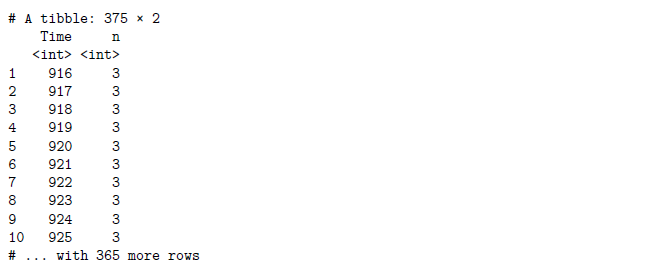rollMean rollMin, and rollMax functions

These functions are part of the timeSeries package. They compute rolling mean, min, and max for a time Series object. The syntax for the rollMean is given as:

rollMean(x, k, na.pad = FALSE)

where, x is a uni or multivariate ‘timeSeries’ object. k is an integer width of the rolling window. na.pad is a logical flag for padding. By default it is FALSE.

Example:

library(timeSeries); library(quantmod);
data = getSymbols("SBIN.NS", src="yahoo", from="2017-01-01", to="2017-01-15", auto.assign=FALSE)
open = timeSeries(data$SBIN.NS.Open) print(head(open, 5))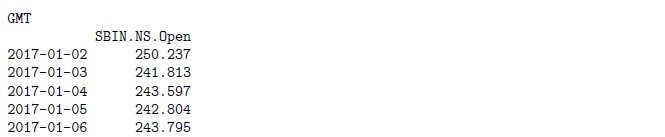rollMean(open, k = 4, na.pad = FALSE)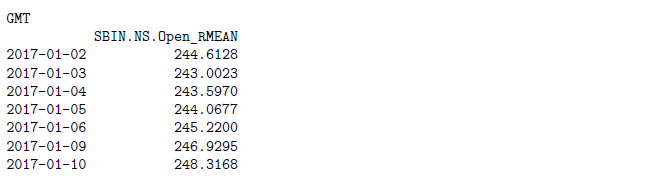The other two functions work in a similar manner as rollMean. Example: library(timeSeries); library(quantmod); data = getSymbols("IOC.NS", src="yahoo", from="2017-01-01", to="2017-01-15", auto.assign=FALSE) close = timeSeries(data$IOC.NS.Close)
rollMax(close, k = 5, na.pad = FALSE)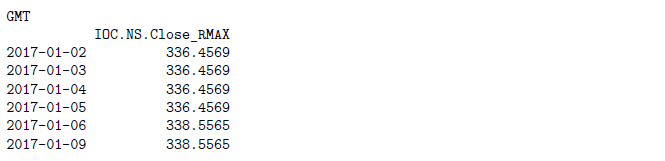### Next Step

We hope you liked this bulletin. In the next weekly bulletin, we will list more interesting ways and methods plus R functions for our readers.

Update

We have noticed that some users are facing challenges while downloading the market data from Yahoo and Google Finance platforms. In case you are looking for an alternative source for market data, you can use Quandl for the same.